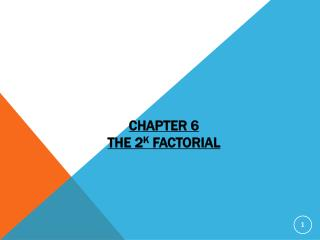DownloadDownload PresentationChapter 6 The 2 k Factorial

# Chapter 6 The 2 k Factorial

Download Presentation## Chapter 6 The 2 k Factorial

- - - - - - - - - - - - - - - - - - - - - - - - - - - E N D - - - - - - - - - - - - - - - - - - - - - - - - - - -
##### Presentation Transcript

1. Chapter 6The 2k Factorial

2. The Essentials of 2-Cubed Designs • Methodology • Cube Plots • Estimating Main Effects • Estimating Interactions (Interaction Tables and Graphs) • Statistical Significance (Effects Probability Plots) • Example With Interactions • A U-Do-It Case Study

3. Methodology Example 2 • Looking For Patterns In The Data To Discover How The Factors Affect The Response, y.

4. Methodology Example 2 - Cube Plot

5. Methodology Example 2 - Estimating the Main Effect of A • Take the Difference of the Average Response for A+ and A- • Eliminate Those Edges of the Cube Where Going from One Cube Corner to Another Involves Changing A from- to + • Average the Corners on the Common Faces and Difference • A=[(70+71+72+73)/4] -[(66+66+68+66)/4] = 71.5-66.5 = 5

6. Methodology Example 2 - Estimating the Main Effect of B

7. Methodology Example 2 - Estimating the Main Effect of C

8. Methodology Example 2 - Signs Table • The Signs of the Main Effects Give The Recipes For the 8 Runs in the Design • Actual Run Corresponds to the Order of the Experimental Runs (Recipes)

9. MethodologyExample 2 - Signs TableUsed to Calculate Effects • To Estimate the Main Effects • Multiply the Response y by the Corresponding Sign Column • Sum the Column • Divide the Sum by the Divisor to Get the Estimated Main Effect • U-Do-It • Calculate the Main Effects Due to B and to C

10. Methodology Example 2 - Estimating the Effect of A Another Way • Average the Differences of A+and A- Over All the Combinations of B and C. • Retain Those Edges of the Cube Where Going from One Cube Corner to Another Involves Changing A from - to + • Difference These Corners and Average • [(72-68)+(71-66)+(73-66) +(70-66)]/4 = 20/4 = 5

11. MethodologyExample 2 - Estimating the Effect of the AB Interaction • Average • The Four Values in the Shaded Corners • The Four Values in the Unshaded Corners • Difference the Averages • [(71+72 +66+66)/4] -[(70+73+66+68)/4]=68.75-69.25=-.5

12. MethodologyExample 2 - Estimating the Effect of the AB Interaction Another Way • The Second Way Shows that the AB Interaction is Comparing the Differences in going from A- to A+ at B- and B+. • If there is a “Significant” Difference, then A and B are said to Interact • [(71-66)+(72-68)-(73-66)-(70-66)]/4 = -0.5 • In this example, there are no significant interactions. Thus, the interpretation is straightforward. • WARNING: When a higher order interaction is “significant,” the direct interpretation of lower order interactions and main effects is misleading.

13. Methodology Example 2 - Signs TableCalculating the Signsand the Effect of Interaction AB

14. MethodologyExample 2 - Signs TableU-Do-It • Calculate the Signs for Interactions AC, BC and ABC • Calculate These Interaction Effects

15. ac=3

16. MethodologyExample 2 - ANOVA Table

17. Methodology Example 2 - Effects Normal Probability Plot

18. MethodologyExample 2 - Discussion • Only the Main Effect A is Significant • Set A Hi to Maximize y • Set A Lo to Minimize y • This Data is Real and Will be Considered in Later Sections • For This Data Minimizing y is the Objective

19. MethodologyExample 2 - Estimating the Response • Since Only the Main Effect A is Significant The Estimated Mean Response (EMR) is given by EMR = y + (Sign of A)(Effect of A)/2 = 69 + (Sign of A)5/2 • For A Lo, EMR = 69 + (-1)(2.5) = 66.5 • For A Hi, EMR = 69 + (+1)(2.5) =71.5

20. MethodologyExample 2 - Estimating the ResponseWhy the One-Half? • The Formula Gives You Just What You Expect: The Average Response at that Level of the A • For A Lo, EMR = 69 + (-1)(2.5) = 66.5 = (66 +66 +66 +68)/4 • For A Hi, EMR = 69 + (+1)(2.5) =71.5 = (70 + 71 + 73 +72)/4

21. II. SummaryKey Ideas • Use Sign Tables to Estimate Effects • Use Probability Plots to Identify Significant Effects • Interaction Tables and Graphs are Used to Analyze Significant Interactions(To be explained later)

22. II. SummaryConcluding Comments • A Main Effect Is Easy To Interpret When There Are No Significant Interactions Involving It • In The Presence of a Significant Higher-Order Interaction, the Lower-Order Interactions and Corresponding Main Effects Are Hard To Interpret by Themselves. (You Still Can Figure Out What to Do, Though) • The Size of the Effects You are Trying to Detect and the Noise of the Process (How Much Variation It Has) Will Dictate How Much Replication Is Needed